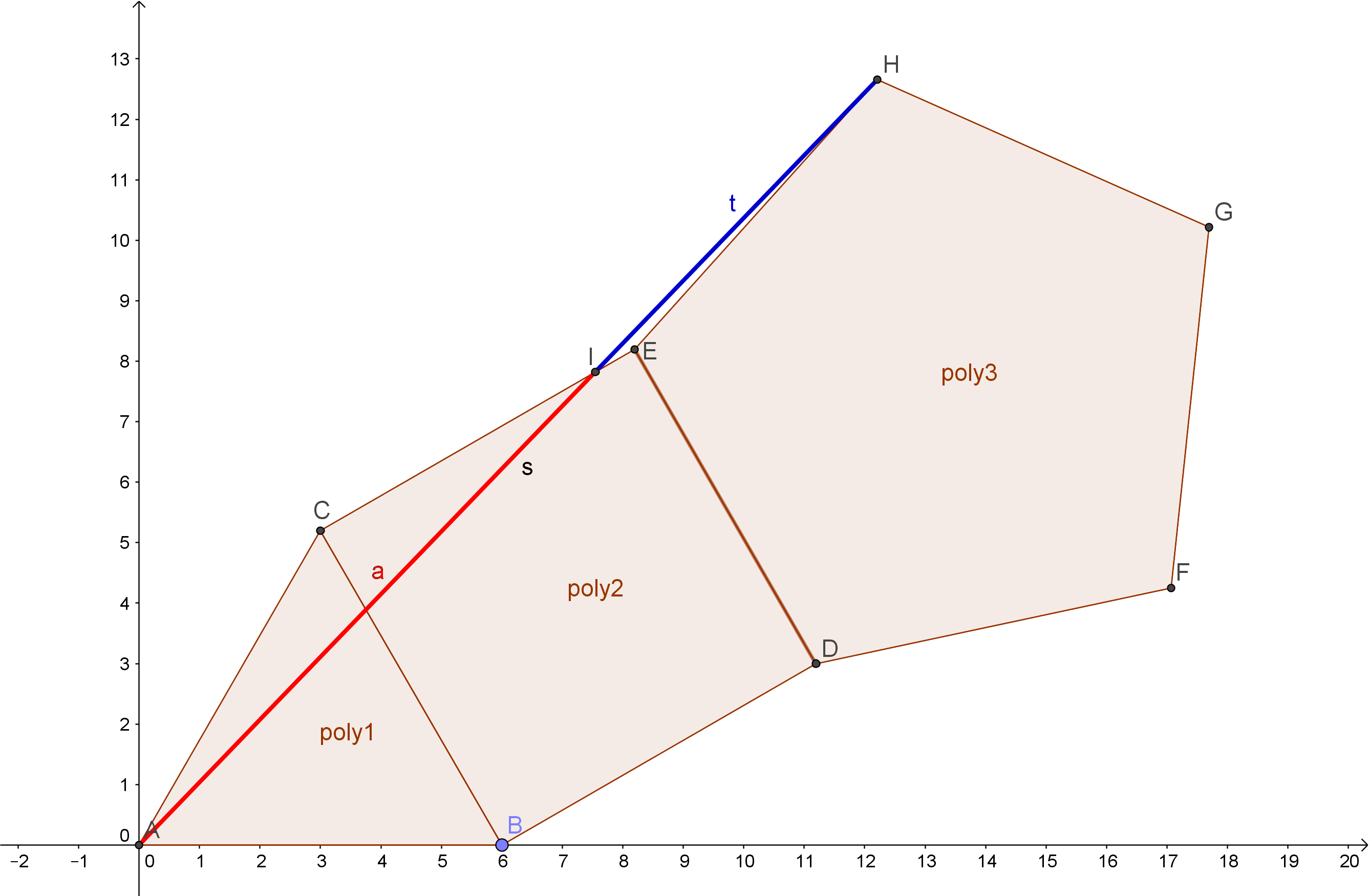# A Klein BottleMs Premise-Conclusion

A Klein bottle is an interesting closed surface that comes up in certain areas of mathematics. After our wonderful librarian at school hooked me up (no pun intended, but I will leave it) with a paper on a knitted version of a Klein bottle, I thought I should really try to crochet one someday. Enter: summer holidays and free time.So, what the heck is it? Unlike a Mobius strip, it doesn’t have an edge, but, like a Mobius strip, it is referred to as a ‘non-orientable’ surface. This means that if you were to run your finger along the entire length of the surface (starting anywhere) you would wind up back where you started, but with your finger on the opposite side of the surface. Trippy.

It’s a cylinder that intersects with itself and then the ends get attached together. Here’s a diagram to help you visualize what…

View original post 156 more words

# The Mathemagician’s RoomIsles of Time

It’s a place full of wonder, strangeness and bizarre beauty: the room – or should I say space – of a “mathematician that’s merely a magician.

It’s a common optical illusion, a kind of tricky paradox. “If our brains are simple enough for us to understand them, then we’d be so simple that we couldn’t.” ~ Quote by Ian Steward (a mathematician)

The floor itself is an impossible figure – a typical symbol for paradoxes…
The spirals on the checkerboard-floor are Fibonacci spirals.

This drawing is mostly about the beauty of Phi Φ, Psi ψ and the Fibonacci-sequence.

The fibonacci sequence
The fibonacci sequence works this way:
1+1 = 2
1+2 = 3
2+3 = 5
3+5 = 8
5+8 = 13
8+13 = 21
13+21 = 34
21+34 = 55
34+55 = 89
55+89…
… and so on…
The sequence then is: 1;1;2;3;5;8;13;21;34;55;89;…….

Let’s play with these numbers!
1/1=1
2/1=2
3/2=1.5

View original post 305 more words

# Platonic Solids and the Golden Ratio

There are five regular polyhedra. These are they: tetrahedron, cube/hexahedron, octahedron, dodecahedron and icosahedron. Here is a short summary in form of a table about the vertices, edges, faces and volumes of these solids:

 Tetrahedron Cube/ Hexahedron Octahedron Dodecahedron Icosahedron v vertices 4 8 6 20 12 e edges 6 12 12 30 30 f faces 4 6 8 12 20 V volume √2 /12*a³ a³ √2 /3*a³ (15+7*√5)/4*a³ (15+5*√5)/12*a³

We could group them into three groups:

• The triangular faced ones {tetrahedron, octahedron, icosahedron}

• The square faced one {cube}

• The pentagon faced one {dodecahedron}

If we put these polygons together like this, we can recognize the golden ratio in it: Here we add a triangle, square and a pentagon together in the following way. Then we connect the point A and H with each other – and exactly where this line crosses the square the golden ratio appears:Source
Also, if you didn’t know about the pentagram, even there appears the golden ratio:Source: Wikipedia

And, two of the platonic solids have the golden ratio in themselves: the icosahderon and the dodecahedron.

Imagine 3 planes put together like this:In a dodechedron the exact same planes can be put into it like this:But if we want the edges of the planes to be on the edges of the dodecahedron, we have to use another ratio:Then the ratio is 1 to phi².